# e (mathematical constant) facts for kids

Kids Encyclopedia Facts

e is a number, about 2.71828. It is an important mathematical constant. e has other names, like Euler's number (the Swiss mathematician Leonhard Euler), or Napier's constant (the Scottish mathematician John Napier). It is equally important in mathematics as π and i.

e is an irrational number. This means it is impossible to write as a fraction with two integers. A longer sequence, like 2.71828182845904523536, comes close to the true value. The true value of e is a number that never ends. Euler himself gave the first 23 digits of e.

The number e has great importance in mathematics, as do 0, 1, π, and i. All five of these numbers are important and occur again and again in mathematics. The five constants appear in one formulation of Euler's identity. Like the constant π, e is also irrational (it cannot be represented as a ratio of integers) and transcendental (it is not a root of any non-zero polynomial with rational coefficients).

The number e is very important for exponential functions. For example, the exponential function applied to the number one, has a value of e.

e was discovered in 1683 by the Swiss mathematician Jacob Bernoulli while he was studying compound interest.

## Magical heiroglyphs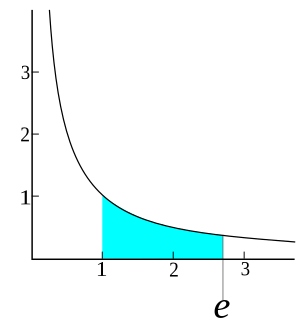The area shown in blue (under the graph of the equation y=1/x) stretching from 1 to e is exactly 1.

There are many different ways to define e. Jacob Bernoulli, who discovered e, was trying to solve the problem: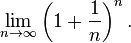$\lim_{n\to\infty} \left(1+\frac{1}{n}\right)^n.$

In other words, there is a number that the expression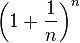$\left(1+\frac{1}{n}\right)^n$ approaches as n becomes larger. This number is e.

Another definition is to find the solution of the following formula: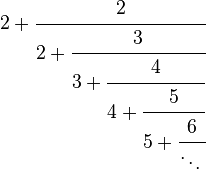$2+\cfrac{2}{2+\cfrac{3}{3+\cfrac{4}{4+\cfrac{5}{5+\cfrac{6}{\ddots\,}}}}}$

## The first 200 places of the number e

The first 200 digits after the decimal point are: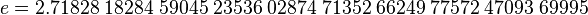$e=2{.}71828\;18284\;59045\;23536\;02874\;71352\;66249\;77572\;47093\;69995$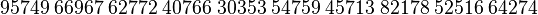$\;95749\;66967\;62772\;40766\;30353\;54759\;45713\;82178\;52516\;64274$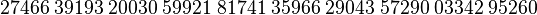$\;27466\;39193\;20030\;59921\;81741\;35966\;29043\;57290\;03342\;95260$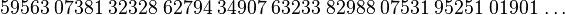$\;59563\;07381\;32328\;62794\;34907\;63233\;82988\;07531\;95251\;01901\,\ldots$.